2021-11-05 10:51:14F5小安

PHPWord

# Questions

1. 用你熟悉的编程语言实现一致性 hash 算法。

2. 编写测试用例测试这个算法，测试 100 万 KV 数据，10 个服务器节点的情况下，计算这些 KV 数据在服务器上分布数量的标准差，以评估算法的存储负载不均衡性。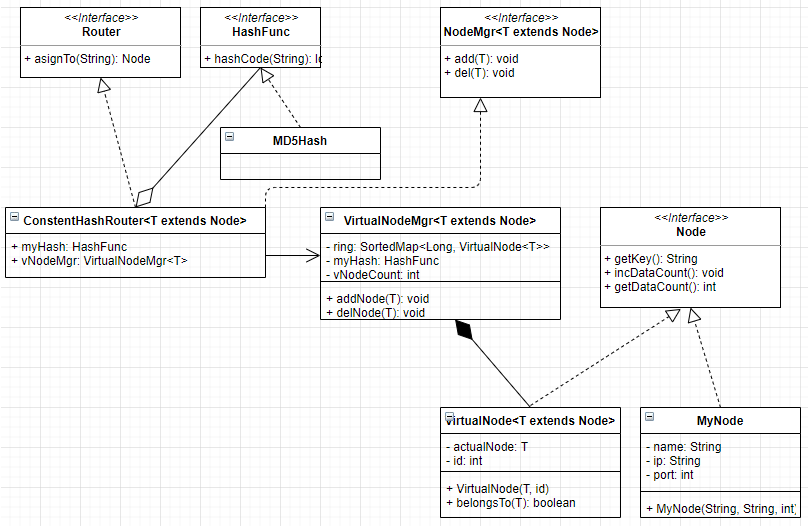``package example.consistent.hash;``public interface HashFunc {``    long hashCode(String key);``}``

``package example.consistent.hash;``import java.security.MessageDigest;``import java.security.NoSuchAlgorithmException;``public class MD5HashFunc implements HashFunc{``    MessageDigest instance;``    public MD5HashFunc() {``        try {``            instance = MessageDigest.getInstance("MD5");``        } catch (NoSuchAlgorithmException e) {``        }``    }``    @Override``    public long hashCode(String key) {``        instance.reset();``        instance.update(key.getBytes());``        byte[] digest = instance.digest();``        long result = 0;``        for (int i = 0; i < 4; i++) {``            result <<= 8;``            result |= ((int) digest[i]) & 0xFF;``        }``        return result;``    }``}``

``package example.consistent.hash;``public interface Node {``    String getKey();``    void incDataCount();``    int getDataCount();``}``

``package example.consistent.hash;``import java.util.Objects;``public class VirtualNode<T extends Node> implements Node {``    private T actualNode;``    private int id;``    protected VirtualNode(T actualNode, int id) {``        if (actualNode == null) {``            throw new NullPointerException("Null actualNode");``        }``        this.actualNode = actualNode;``        this.id = id;``    }``    @Override``    public String getKey() {``        return String.format("%s#%s", this.actualNode.getKey(), this.id);``    }``    @Override``    public void incDataCount() {``        throw new UnsupportedOperationException();``    }``    @Override``    public int getDataCount() {``        throw new UnsupportedOperationException();``    }``    protected boolean belongsTo(T oneNode) {``        if (oneNode == null) {``            throw new NullPointerException();``        }``        return Objects.equals(this.actualNode.getKey(), oneNode.getKey());``    }``    protected T getActualNode() {``        return actualNode;``    }``}``

``package example.consistent.hash;``public class MyNode implements Node {``    private String name;``    private String ip;``    private int port;``    private AtomicInteger dataCount = new AtomicInteger();``    public MyNode(String name, String ip, int port) {``        this.name = name;``        this.ip = ip;``        this.port = port;``    }``    @Override``    public String getKey() {``        return String.format("%s#%s#%s", name, ip, port);``    }``    @Override``    public void incDataCount() {``        dataCount.incrementAndGet();``    }``    @Override``    public int getDataCount() {``        return dataCount.get();``    }``}``

``package example.consistent.hash;``import java.util.Iterator;``import java.util.Objects;``import java.util.SortedMap;``import java.util.TreeMap;``public class VirtualNodeMgr<T extends Node> implements Router{``    private SortedMap<Long, VirtualNode<T>> ring = new TreeMap<>();``    private HashFunc hash;``    private int vNodeCountPerActualNode;``    protected VirtualNodeMgr(HashFunc hash, int vNodeCountPerActualNode) {``        if (hash == null) {``            throw new NullPointerException(HashFunc.class.getSimpleName());``        }``        this.hash = hash;``        if (vNodeCountPerActualNode <= 0) {``            throw new IllegalArgumentException("The number of virtual nodes for each actual node is equal to or smaller than zero!");``        }``        this.vNodeCountPerActualNode = vNodeCountPerActualNode;``    }``    @Override``    public Node asignTo(String key) {``        if (ring.isEmpty()) {``            return null;``        }``        Long hashVal = hash.hashCode(key);``        SortedMap<Long,VirtualNode<T>> tailMap = ring.tailMap(hashVal);``        Long nodeHashVal = !tailMap.isEmpty() ? tailMap.firstKey() : ring.firstKey();``        Node result = null;``        if (ring.containsKey(nodeHashVal)) {``            result = ring.get(nodeHashVal).getActualNode();``            result.incDataCount();``        }``        return result;``    }``    protected void addNode(T oneNode) {;``        int existingVNodeCount = getExistingVNodeCount(oneNode);``        for (int i = 0; i < vNodeCountPerActualNode; i++) {``            VirtualNode<T> vNode = new VirtualNode<>(oneNode, i + existingVNodeCount);``            ring.put(hash.hashCode(vNode.getKey()), vNode);``        }``    }``    protected void delNode(T oneNode) {``        if (Objects.isNull(oneNode)) {``            throw new NullPointerException();``        }``        Iterator<Long> it = ring.keySet().iterator();``        while (it.hasNext()) {``            VirtualNode<T> virtualNode = ring.get(it.next());``            if (virtualNode.belongsTo(oneNode)) {``                it.remove();``            }``        }``    }``    private int getExistingVNodeCount(T pNode) {``        int replicas = 0;``        for (VirtualNode<T> vNode : ring.values()) {``            if (vNode.belongsTo(pNode)) {``                replicas++;``            }``        }``        return replicas;``    }``}``

``package example.consistent.hash;``public interface Router {``    Node asignTo(String key);``}``

``package example.consistent.hash;``public interface NodeMgr<T extends Node> {``    void add(T oneNode);``    void del(T oneNode);``}``

``package example.consistent.hash;``public class VirtualConsistentHashRouter implements Router, NodeMgr{``    private HashFunc myHash = new MD5HashFunc();``    private VirtualNodeMgr mgr;``    public VirtualConsistentHashRouter(HashFunc yourHash, int vNodeCountPerActualNode) {``        if (yourHash != null) {``            myHash = yourHash;``        }``        mgr = new VirtualNodeMgr(myHash, vNodeCountPerActualNode);``    }``    public VirtualConsistentHashRouter(){``        mgr = new VirtualNodeMgr(myHash, 150);``    }``    @Override``    public Node asignTo(String key) {``        return mgr.asignTo(key);``    }``    @Override``    public void add(Node oneNode) {``        mgr.addNode(oneNode);``    }``    @Override``    public void del(Node oneNode) {``        mgr.delNode(oneNode);``    }``}``

## 100 万测试数据模拟

``package example.consistent.hash;``import java.util.HashMap;``import java.util.Map;``import java.util.UUID;``public class Test {``    private static final int DATA_COUNT = 1000000;``    public static void main(String[] args) {``        MyNode[] nodes = {new MyNode("Jiangsu", "192.168.0.1", 8440),``                new MyNode("Shandong", "192.168.0.2", 8441),``                new MyNode("Zhejiang", "192.168.0.3", 8442),``                new MyNode("Hainan", "192.168.0.4", 8443),``                new MyNode("Henan", "192.168.0.5", 8444),``                new MyNode("Hebei", "192.168.0.6", 8445),``                new MyNode("Shanxi", "192.168.0.7", 8446),``                new MyNode("Shangxi", "192.168.0.8", 8447),``                new MyNode("Shandong", "192.168.0.9", 8448),``                new MyNode("Gansu", "192.168.0.10", 8449)};``        VirtualConsistentHashRouter router = new VirtualConsistentHashRouter();``        for (MyNode oneNoe : nodes) {``            router.add(oneNoe);``        }``        long startT = System.currentTimeMillis();``        Map<String, Node> data = new HashMap<>(DATA_COUNT);``        String key = null;``        Node serviceNode = null;``        for (int i = 0; i < DATA_COUNT; i++) {``            key = UUID.randomUUID().toString();``            serviceNode = router.asignTo(key);``            data.put(key, serviceNode);``        }``        long endT = System.currentTimeMillis();``        int totalDataCount = 0;``        for ( MyNode oneNode : nodes) {``            totalDataCount += oneNode.getDataCount();``        }``        int avgDataCountPerNode = totalDataCount / nodes.length;``        int tmpSum = 0;``        for ( MyNode oneNode : nodes) {``            tmpSum += Math.pow(oneNode.getDataCount() - avgDataCountPerNode, 2);``        }``        int tmpAvg = tmpSum / nodes.length;``        System.out.println(String.format("采用虚拟一致性hash算法分布%s个数据耗时：%sms", DATA_COUNT, endT - startT));``        System.out.println(String.format("平均每个节点缓存数据个数：%s 总体标准差：%s", avgDataCountPerNode, Math.sqrt(tmpAvg)));``    }``

## 运行结果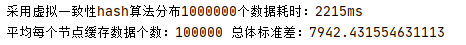# Summary

## 2、合理使用缓存

### 频繁修改的数据：

LRU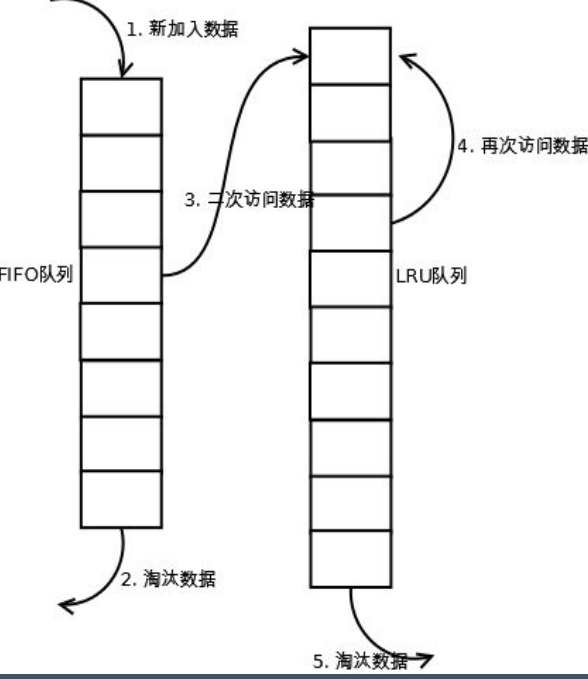### 数据不一致与脏读：

“计算机科学中只有三件事最困难:缓存失效，命名事物，计数错误。”

——Phil Karlton

## 3、缓存的关键指标

### 缓存命中率

•缓存是否有效依赖于能多少次重用同一个缓存响应业务请求，这个度量指标被称作缓存命中

•如果查询一个缓存，十次查询九次能够得到正确结果，那么它的命中率是 90%。

### 影响缓存命中率的主要指标

#### • 缓存对象生存时间

• 代理缓存，反向代理缓存，CDN 缓存都是通读缓存。

• 通读缓存给客户端返回缓存资源，并在请求未命中缓存时获取实际数据。

• 客户端连接的是通读缓存而不是生成响应的原始服务器。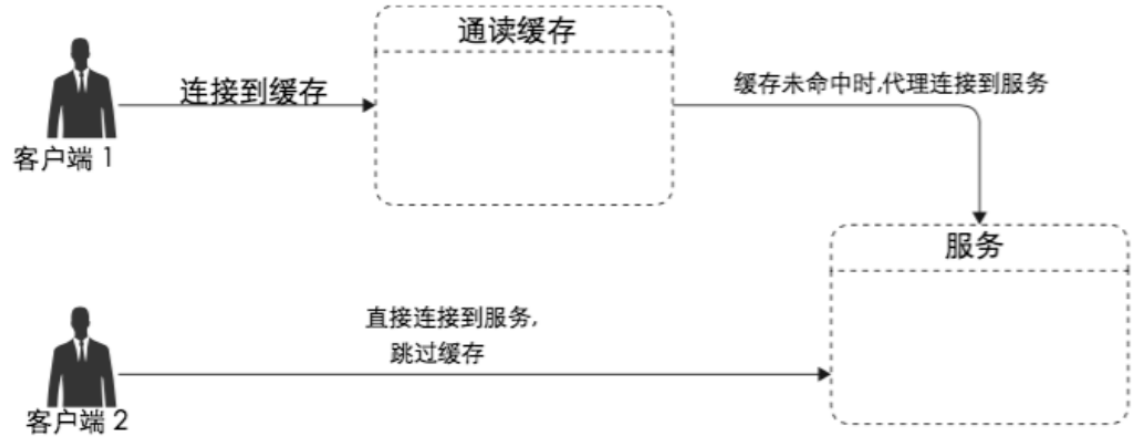## 5、旁路缓存（cache-aside）

• 对象缓存是一种旁路缓存，旁路缓存通常是一个独立的键值对（key-value）存储。

• 应用代码通常会询问对象缓存需要的对象是否存在，如果存在，它会获取并使用缓存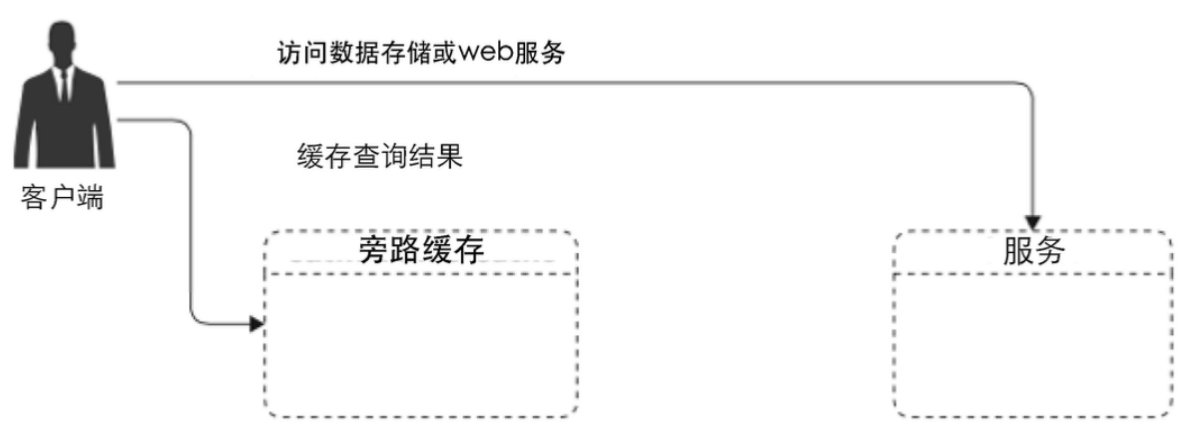## 6、路由算法

### 6.2 一致性 hash 算法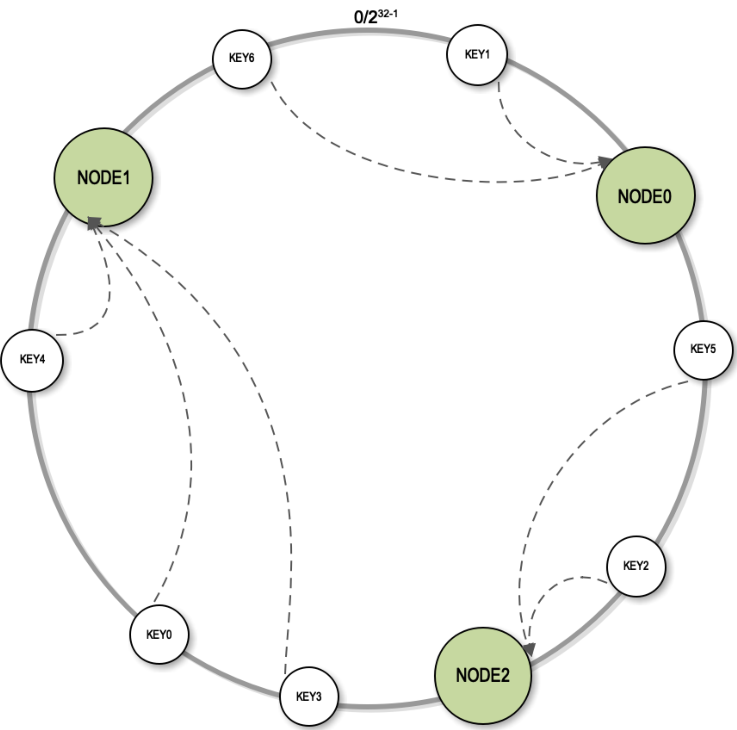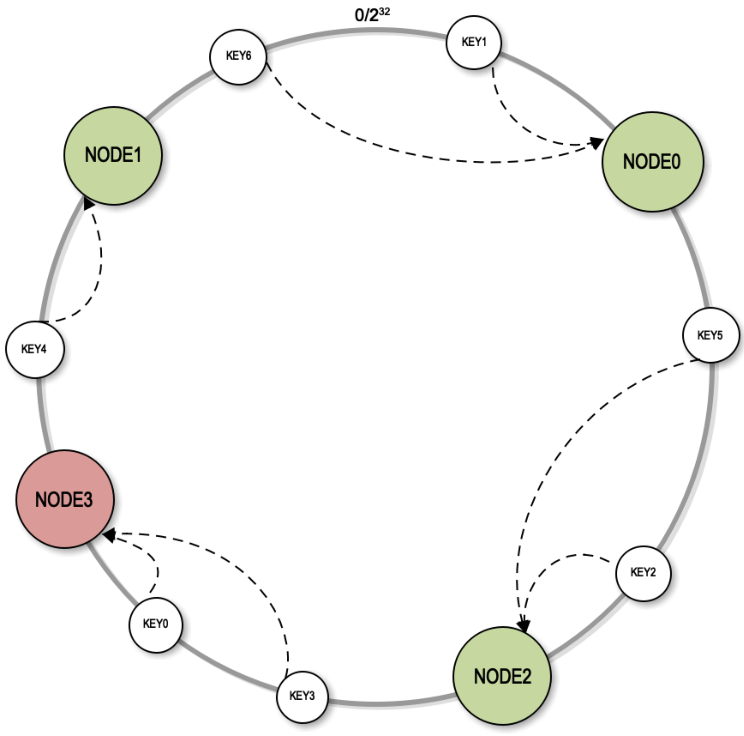### 6.3 基于虚拟节点的一致性 Hash 算法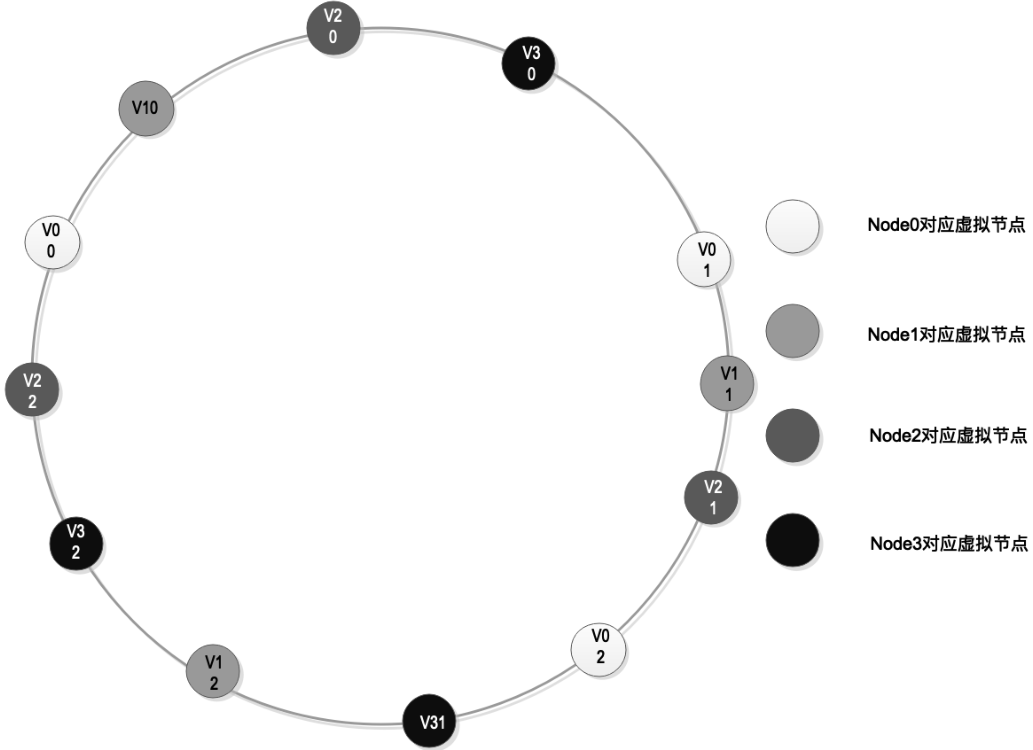## 7、EDA 事件驱动架构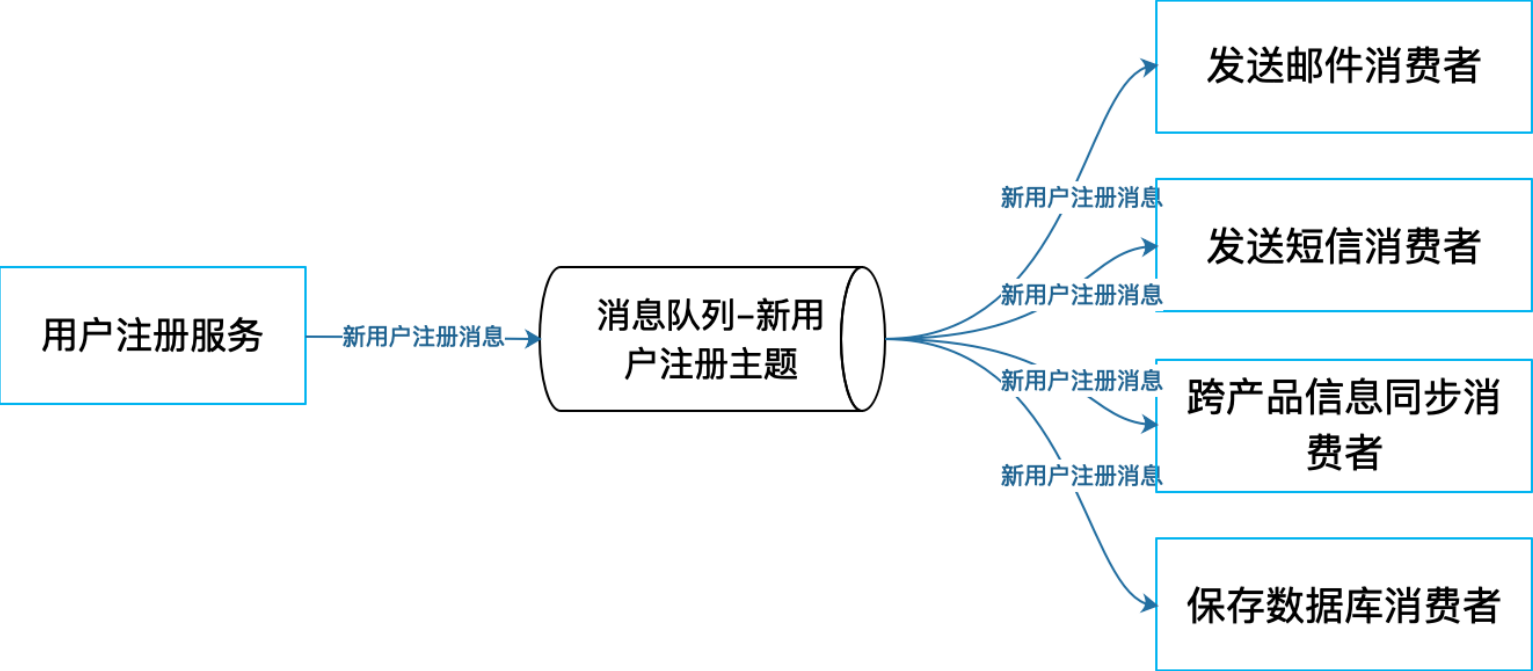## 8、常见的 MQ 开源组件活跃度：

kafka(Scala)>RocketMQ(Java)>RabbitMQ(Erlang)>ActiveMQ(Java)

F5社区好文推荐：F5 分布式数据库增强型架构F5小安

2022-01-14 09:11:0850F5小安

2021-11-22 13:19:2480F5小安

2021-11-18 17:45:2293

© 2019 F5 Networks, Inc. 版权所有。京ICP备16013763号-1